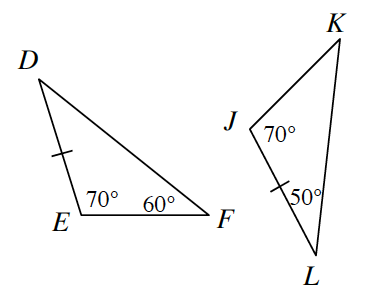### Home > CCG > Chapter 6 > Lesson 6.1.3 > Problem6-26

6-26.Examine the two triangles at right.

1. Are the triangles congruent? Justify your conclusion. If they are congruent, complete the congruence statement $ΔDEF\;\text{_____}$.

Use the Triangle Angle Sum Theorem to help find the missing angles, then determine whether or not the triangles are similar.

2. What series of transformation(s) are needed to change $ΔDEF$ to $ΔLJK$?

Reflection and rotation.

3. If $DE=4$ units, find $KL$.

Use the Law of Sines to solve for $KL$.